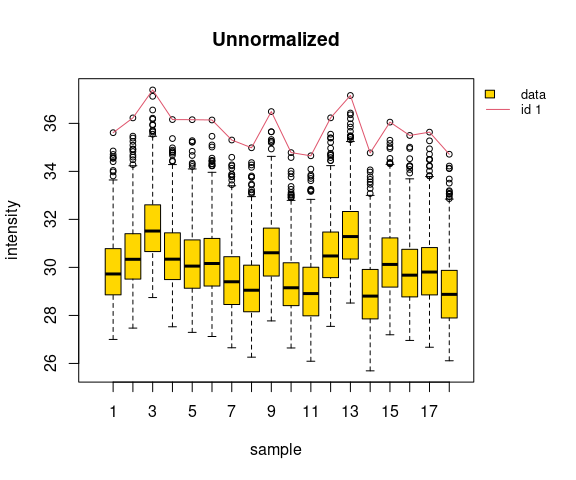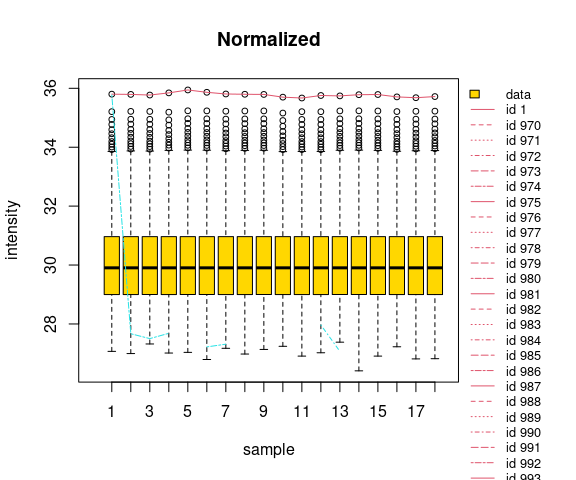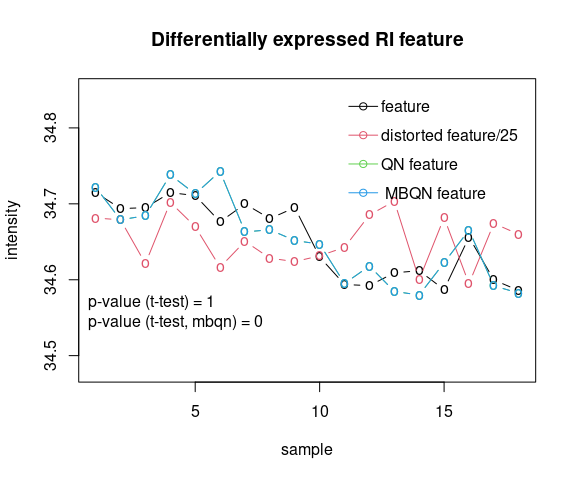# MBQN vignette

This package contains a modified quantile normalization (QN) for preprocessing and analysis of omics or other matrix-like organized data with intensity values biased by global, columnwise distortions of intensity mean and scale. The modification balances the mean intensity of features (rows) which are rank invariant (RI) or nearly rank invariant (NRI) across samples (columns) before quantile normalization . This helps to prevent an over-correction of the intensity profiles of RI and NRI features by classical QN and therefore supports the reduction of systematics in downstream analyses. Additional package functions help to detect, identify, and visualize potential RI or NRI features in the data and demonstrate the use of the modification.

## Installation

To install this package, you need R version >= 3.6.

For installation from Bioconductor run in R:

# if (!requireNamespace("BiocManager", quietly = TRUE))
#    install.packages("BiocManager")
BiocManager::install("MBQN")

## Dependencies

The core of the MBQN package uses normalizeQuantiles() from the package limma , available at https://bioconductor.org/packages/release/bioc/html/limma.html, for computation of the quantile normalization. Optionally, normalize.quantiles() from the package preprocessCore , available at https://bioconductor.org/packages/release/bioc/html/preprocessCore.html, can be used.

To install these packages in R run:

# if (!requireNamespace("BiocManager", quietly = TRUE))
#    install.packages("BiocManager")
# BiocManager::install(pkgs = c("preprocessCore","limma", "SummarizedExperiment"))

## Usage

The package provides two basic functions: mbqn() applies QN or mean/median-balanced quantile normalization (MBQN) to a matrix. mbqnNRI() applies quantile normalization and mean/median-balanced quantile normalization only to selected NRI and RI features, specified by a threshold or manually. The input matrix may contain NAs. To run one of these functions you will need to provide an input matrix (see Examples). The argument FUN is used to select between classical quantile normalization (default), and mean or median balanced quantile normalization. The function mbqnGetNRIfeatures() and mbqnPlotRI() can be used to check a data matrix for RI or NRI features. They provide a list of potential RI/NRI features together with their rank invariance frequency and a graphical output.

## Examples

Example 1: Generate a distorted omics-like matrix of log2-transformed intensities with missing values and a single rank invariant feature:

## basic example
library("MBQN")
set.seed(1234)
# data generation
mtx <- mbqnSimuData("omics.dep")
# data distortion
mtx <- mbqnSimuDistortion(mtx)$x.mod plot.new() mbqnBoxplot(mtx, irow = 1, main = "Unnormalized") ## Warning in (function (z, notch = FALSE, width = NULL, varwidth = FALSE, : ## Duplicated argument main = "Unnormalized" is disregardedFig. 1 Boxplot of the unnormalized, distorted intensity data matrix. The first feature is an RI feature (red line). It has maximum intensity for each sample! Apply check for rank invariant (RI) or nearly rank invariant (NRI) features to the data matrix and visualize result: res <- mbqnGetNRIfeatures(mtx, low_thr = 0.5) ## Caution: There might be multiple RI/NRI features! ## Maximum frequency of RI/NRI feature(s): 100 % Apply quantile normalization with and without balancing the RI feature and compare the intensity features: plot.new() mbqn.mtx <- mbqnNRI(x = mtx, FUN = median, verbose = FALSE) # MBQN qn.mtx <- mbqnNRI(x = mtx, FUN = NULL, verbose = FALSE) # QN mbqnBoxplot(mbqn.mtx, irow = res$ip, vals = data.frame(QN = qn.mtx[res$ip,]), main = "Normalized") ## Warning in (function (z, notch = FALSE, width = NULL, varwidth = FALSE, : ## Duplicated argument main = "Normalized" is disregardedFig. 2 Quantile normalized intensities with balanced and unbalanced normalized RI feature. Classical quantile normalization suppresses any intensity variation of the RI feature, while the MBQN preserves its variation while reducing systematic batch effects! Example 2: Visualize the effect of normalization on rank mixing and rank invariant intensity features by comparing the intensity distribution of unnormalized, quantile and mean/median-balanced quantile normalized data on a matrix where rows represent features, e.g. of protein abundances/intensities, and columns represent samples. ## basic example library("MBQN") set.seed(1234) mtx <- mbqnSimuData("omics.dep") # Alternatively: mtx <- matrix( # c(5,2,3,NA,2,4,1,4,2,3,1,4,6,NA,1,3,NA,1,4,3,NA,1,2,3),ncol=4) Perform QN, median balanced QN, and QN with median balanced NRI feature. qn.mtx <- mbqn(mtx,FUN=NULL, verbose = FALSE) mbqn.mtx <- mbqn(mtx,FUN = "median", verbose = FALSE) qn.nri.mtx <- mbqnNRI(mtx,FUN = "median", low_thr = 0.5, verbose = FALSE) Check saturation i.e. for rank invariance. res <- mbqnGetNRIfeatures(mtx, low_thr = 0.5) ## Caution: There might be multiple RI/NRI features! ## Maximum frequency of RI/NRI feature(s): 100 % # Maximum frequency of RI/NRI feature(s): 100 % Example 3: Apply a two-sided t-test before and after application of different normalizations to a simulated, differentially expressed and distorted RI feature. The feature is obtained from a simulated dataset where each sample is distorted in mean and scale. #plot.new() mtx <- mbqnSimuData("omics.dep", show.fig = FALSE) mod.mtx <- mbqnSimuDistortion(mtx, s.mean = 0.05, s.scale = 0.01) mtx2 <- mod.mtx mod.mtx <- mod.mtx$x.mod

res <- mbqnGetNRIfeatures(mod.mtx, low_thr = 0.5)
## Caution: There might be multiple RI/NRI features!
## Maximum frequency of RI/NRI feature(s):  100 %
# undistorted feature
feature1 <- mtx[1,]
# distorted feature
feature1mod = mod.mtx[1,]
# feature after normalization
qn.feature1 = mbqn(mod.mtx, verbose = FALSE)[1,]
qn.mtx = mbqn(mod.mtx,verbose = FALSE)

mbqn.mtx = mbqn(mod.mtx, FUN = "mean",verbose = FALSE)
mbqn.feature1 = mbqn(mod.mtx, FUN = "mean",verbose = FALSE)[1,]

Apply t-test:

# undistorted feature
ttest.res0 <- t.test(feature1[seq_len(9)], feature1[c(10:18)],
var.equal =TRUE)
# distorted feature
ttest.res1 <- t.test(feature1mod[seq_len(9)], feature1mod[c(10:18)],
var.equal =TRUE)
# mbqn normalized distorted feature
ttest.res <- t.test(mbqn.feature1[seq_len(9)], mbqn.feature1[c(10:18)],
var.equal =TRUE)

Compare QN, MBQN and original feature.

plot.new()
matplot(t(rbind(feature1 = feature1,
mod.feature1 = (feature1mod-mean(feature1mod))/25+mean(feature1),
qn.feature1 = (qn.feature1-mean(qn.feature1))+mean(feature1),
mbqn.feature1 = (
mbqn.feature1-mean(mbqn.feature1))+mean(feature1))),
type = "b", lty = c(1,1,1), pch = "o",
ylab = "intensity",
xlab = "sample",
main = "Differentially expressed RI feature",
ylim = c(34.48,34.85))
legend(x=11,y= 34.86, legend = c("feature","distorted feature/25" ,
"QN feature", " MBQN feature"),pch = 1,
col = c(1,2,3,4), lty= c(1,1,1,1), bty = "n", y.intersp = 1.5,
x.intersp = 0.2)
legend(x = .1, y = 34.6,
legend = paste("p-value (t-test) =",round(ttest.res1$p.value,2), "\np-value (t-test, mbqn) =", round(ttest.res$p.value,4)),
bty = "n", x.intersp = 0)Fig. 3

if (ttest.res$p.value<0.05) message("H0 (=equal mean) is rejected!") ## H0 (=equal mean) is rejected! # print(mtx2$x.mod)
# print(mtx2$mx.offset) # print(mtx2$mx.scale)
print(paste("ttest.undistorted =",ttest.res0)) 
##   "ttest.undistorted = c(t = 10.0729609396921)"
##   "ttest.undistorted = c(df = 16)"
##   "ttest.undistorted = 2.48237068197741e-08"
##   "ttest.undistorted = c(0.0715256392245863, 0.109656286984875)"
##   "ttest.undistorted = c(mean of x = 34.6978790054118, mean of y = 34.607288042307)"
##   "ttest.undistorted = c(difference in means = 0)"
##   "ttest.undistorted = 0.00899347904227051"
##   "ttest.undistorted = two.sided"
##   "ttest.undistorted =  Two Sample t-test"
##  "ttest.undistorted = feature1[seq_len(9)] and feature1[c(10:18)]"
print(paste("ttest.distorted =", ttest.res1))
##   "ttest.distorted = c(t = -0.00510882259762921)"
##   "ttest.distorted = c(df = 16)"
##   "ttest.distorted = 0.995986923648603"
##   "ttest.distorted = c(-0.877522816321726, 0.873303448226306)"
##   "ttest.distorted = c(mean of x = 36.1994057861729, mean of y = 36.2015154702206)"
##   "ttest.distorted = c(difference in means = 0)"
##   "ttest.distorted = 0.412949169283929"
##   "ttest.distorted = two.sided"
##   "ttest.distorted =  Two Sample t-test"
##  "ttest.distorted = feature1mod[seq_len(9)] and feature1mod[c(10:18)]"
print(paste("ttest.mbqndistorted =", ttest.res))
##   "ttest.mbqndistorted = c(t = 5.61617252905213)"
##   "ttest.mbqndistorted = c(df = 16)"
##   "ttest.mbqndistorted = 3.86388948816137e-05"
##   "ttest.mbqndistorted = c(0.0534488302470274, 0.118264504238475)"
##   "ttest.mbqndistorted = c(mean of x = 36.2430019696062, mean of y = 36.1571453023635)"
##   "ttest.mbqndistorted = c(difference in means = 0)"
##   "ttest.mbqndistorted = 0.0152873984548409"
##   "ttest.mbqndistorted = two.sided"
##   "ttest.mbqndistorted =  Two Sample t-test"
##  "ttest.mbqndistorted = mbqn.feature1[seq_len(9)] and mbqn.feature1[c(10:18)]"